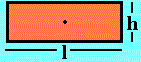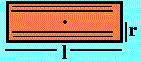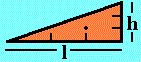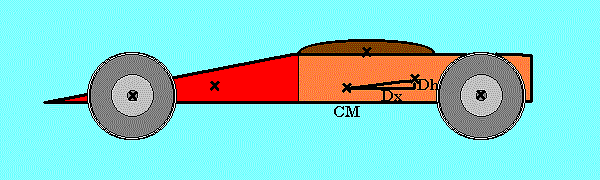Grand Prix Racing - The Science of Fast Pinewood Cars

# Look Up to the Hills

Rotations manifest themselves in many unexpected ways. Often vibrations are rotations. Zig-zag movements may include rotations that switch direction long before completing a full cycle. So it is with your car's body, not only do wheels roll, but to some extent, so does the car body. Unlike a wheel, the "axle" it rotates around is not visible. It doesn't even need to be "in" the car. It's the car's center of mass!

This rotation of the car body as it transitions from the ramp of the race track to the flat is a rather subtle event. The axis of this rotation lies along the width of the car, its pitch axis. There is only a partial rotation. Perhaps it has escaped your notice until now. Never-the-less, it is as interesting a feature of your car's behavior as any other and it uses up a bit of energy.

Another reason to know what the moment of inertia of your car is is to be able to determine how big a bump in the track could cause your car to jump up.

Below, a model for determining the moment of inertia of your car is presented. You can use the worksheet to evaluate the model to get the moment of inertia for your car. Using the evaluation for one car from the example below, we will see how much energy is being used and what it means in terms of forces.

## A model for a car's moment of inertia

In order to estimate your car's moment of inertia about the pitch axis, the car is broken up into smaller parts. These parts include the wheels, various parts of the body and any decorations attached to it. The body is the most difficult part to divide up. There are many shapes for which a moment of inertia formula has been determined. Some listed below are simple shapes that our Grand Prix cars can be divided into. Success of this model depends on how well your car can be broken up into these shapes and how good your measurements are from the center of mass of your car to the center of mass of each part as described below.

Here is a list of shapes and formulas for determining the moment of inertia and center of mass for some three dimensional shapes. Each shape's volume is assumed to have uniform density so that its center of mass is in its geometric center. Note that the width dimension is not drawn. Only the side having length and height is drawn. The center of mass location is along the length and height axies of the shape. For each shape, the axis of rotation passes through it's center of mass and is at right angles to the side drawn. The center of mass locations (cmh,cmx) are measured from the lower left of the shape drawing.

SHAPE NAME cmh cmx MOMENT OF INERTIABlock h/2 l/2 m(h2+l2)/12Cylinder r l/2 m(3r2+l2)/12Rod r r mr2/2Right Wedge h/3 2l/3 m(4h2+l2)/72

The shapes in the table are matched to your car to determine the parts to divide it into. This is not easy, nor exact. The better it is done, the more acurate the result will be. But don't worry if it is not perfect.

Pinewood has a weight density of about 0.28 ounces per cubic inch. So you can measure the volume of each of your shapes and estimate its weight by multiplying its volume by 0.28 oz/in3 or just weigh the thing. To get mass, we divide by 386.088 in/s2.

Here's an example for a car that looks like this:This car is broken up into: a wedge, the front; a block, the back; a cylinder, the cockpit on top; two axle rod pairs; and two pairs of wheels. Here's what it looks like on paper. Note the wheels are dealt with separately in the next chart. CMh = 0.7 inches and CMx = 4.4 inches

We'll use AWANA kit axles so we can get the weight (0.0416 oz each) and moment of inertia. Now, we can make a chart like:

Part SHAPE m ozs2/in h (or r) in l in cmh in cmx in MOMENT OF INERTIA ozins2
_Front_Axle_ _Rod____ _0.00022 _0.045__ _N/A____ _0.045__ _0.045__ _0.00000022
_Rear_Axle__ _Rod____ _0.00022 _0.045__ _N/A____ _0.045__ _0.045__ _0.00000022
_Front__ _Wedge____ _0.00167 _0.75___ _3.5____ _0.25___ _2.333__ _0.00033632
_Back___ _Block____ _0.00333 _0.75___ _3.5____ _0.375__ _1.75___ _0.00355547
_Top____ _Cylinder_ _0.00028 _0.25___ _2.0____ _0.25___ _1.0____ _0.00009938
_Weight_ _2.9 oz___ _0.00658 _N/A____ _N/A____ _N/A____ _N/A____ _N/A_______

We'll use AWANA kit wheels so we can get the weight and moment of inertia. Now, we can make a chart like:

Wheel Pair m ozs2/in MOMENT OF INERTIA ozins2
Front _0.00043 _0.00000096
Rear _0.00043 _0.00000096

A measurement in height and length will be needed from the center of mass of each part (cmh,cmx) to the center of mass of the car (CMh,CMx). These measurements are labeled Dh and Dx. They are used to get the moment of inertia of each part about the center of mass of the car. The physical theorem that allows us to do this is called the parallel axis theorem. The final chart looks like this:

Part m ozs2/in MOMENT OF INERTIA ozins2 Dh in Dx in I+m(Dh2+Dx2)
_Front_Wheels_ _0.00043 _0.00000096 _-0.1___ _-3.25__ _0.004547135
_Rear_Wheels__ _0.00043 _0.00000096 _-0.1___ _2.0____ _0.00172526
_Front_Axles__ _0.00022 _0.00000022 _-0.1___ _-3.25__ _0.00232617
_Rear_Axles___ _0.00022 _0.00000022 _-0.1___ _2.0____ _0.00088242
_Front________ _0.00167 _0.00033632 _0.05___ _-2.067_ _0.00747555
_Back_________ _0.00333 _0.00355547 _0.175__ _0.85___ _0.00606338
_Top__________ _0.00028 _0.00009938 _0.55___ _0.1___ _0.00018688
_Free_Weight__ _0.00658 _N/A_______ _0.0____ _0.0___ _0.0____
TOTAL _0.01250 _0.02320680

Now, we total up the moment of inertia column to get the moment of inertia for the whole car about its pitch axis through its center of mass.

## How much energy?

The amount of energy in this rotation can be studied by noting the usual expression for the energy of a rotating object,

T = Iw2/2 where T represents rotational kinetic energy, I is the moment of inertia and w is the rotational rate of the car.

From the above example, we set I to 0.02320680 ozins2. For w, consider the abrupt transition of the simple closed race model and note that a car will pass over it from front wheel to rear in about 4 hundredths of a second at typical speeds. Thus the car body rotates through 21.2 degrees in 0.04 seconds. Converting degrees to radians, we get w = 0.369/0.04 = 9.225 radians per second.

T = 0.02320680(9.225)2/2 = 0.99 ozin.

The total available potential energy at the start of a race is about 200 ozin. So the rotational energy of the body is about 0.99/200 = 0.5 percent of that available! That's pretty small. And that's as big as it gets! On a smooth transition, the same rotation angle is moved through, but it happens over a much longer time period, perhaps a second even. Then the rotation rate, w, would only be 0.369 radians per second and T becomes, 0.0016 ozin!

## How much Force?

Starting with the energy relation, we can take the time derivative, then divide out velocity to get the force of rotation.

dE/dt = I(dw2/dt)/2 = Iwdw/dt = Iw(dw/dy)(dy/dt) = Iwvdw/dy

When the v is divided out, this becomes the "force" of body rotation in the equations of motion: Iwdw/dy. dw/dy is the change in the rate of body rotation with respect to the change in distance along the track. This is 0 on the ramp and flat. On the transition, it begins positive, then dips past zero to negative values before returning to zero on the flat. The energy stored during the positive thrust is expressed as force on the axles during the negative or deccelerating part of the change in rotation rate.

For the abrupt transition, dw/dy is about (9.225 rad/s)/(6 in) = 1.537 rad/ins. Because the change in rotation rate is not constant, this is a "ball park" estimate probably close in terms of the order of magnitude of the answer.

Iwdw/dy = 0.02320680(9.225)1.537 = 0.3290 oz.

On the smooth transition, dw/dy could be about 0.369/120 = 0.003 rad/ins

So, Iwdw/dy = 0.02320680(0.369)0.003 = 0.000026 oz.

Of course, these are just rough estimates, but in general they show that your typical smooth track race will not be greatly affected by the car body rotation energy loss.

 [Pit Area] [Title Page]